In :
%pylab inline

Populating the interactive namespace from numpy and matplotlib


# SMEDG Python Presentation¶

### 27 August 2015¶

#### By Rupert Osborn¶

Consulting Geologist at H&S Consultants

This IPython Notebook was presented at a SMEDG presentation. The idea behind this notebook is to introduce geologists to some of the benefits of using Python for data analysis. It is recommended to copy and paste the bits of code that you want to use into your own notebook.

In my experience geolgists tend to use MS Excel for data analysis but Python makes it much easier to dig deeper into data and therefore identify relationships that may have been missed. Python code also tends to be more reusable allowing for easier automation of repeatable tasks.

### Why do we use NumPy?¶

The core Python package gives us all the tools we need to operate on numbers. Here is an example where we do a simple calculation on numbers 1 to 2,500,000

In :
import math as math

In :
loops = 2500000
a = range(1, loops)

def math_function(x):
return(3 * math.log(x) + math.cos(x) ** 2)

%timeit r = [math_function(x) for x in a]

1 loops, best of 3: 2.71 s per loop


It worked but it was too slow. Let's try it using NumPy

In :
import numpy as np

In :
def numpy_function(array):
return(3 * np.log(array) + np.cos(array)**2)

In :
a = np.arange(1, loops)
%timeit r = numpy_function(a)

10 loops, best of 3: 179 ms per loop


Much faster! Around 15 times faster. There are faster ways to achieve calculations if you're really interested in speed but unless you're writing serious code or programs I wouldn't bother. If you are concerned or some bits of your code are taking too long to run check out numexpr and/or Cython. Here's a quick example with numexpr:

In :
import numexpr as ne
numexpr_func = '3 * log(a) + cos(a) ** 2'
%timeit r = ne.evaluate(numexpr_func)

100 loops, best of 3: 18.7 ms per loop


Another major reason why we want to use numpy arrays is that we can operate on them easily. Below is an example comparing what hapeens if we multiply a python list by two:

In :
python_list = [1, 2, 3, 4, 5]
python_list*2

Out:
[1, 2, 3, 4, 5, 1, 2, 3, 4, 5]

Compared to what happens if we multiply a numpy array by two:

In :
numpy_array = np.array([1, 2, 3, 4, 5])
numpy_array*2

Out:
array([ 2,  4,  6,  8, 10])

## Why do we use Pandas?¶

First of all Pandas makes it easy to import data from a variety of files including csv and MS Excel

To load a csv file into memory you'll need to tell it the file path. A quick way do do this is go to the file in Windows Explorer, press Ctrl+ right-click and then select "Copy as path". Then you can just paste the whole file path as I've done below.

In :
file_path = "C:\Projects\Python Presentation\Data\ExampleData.csv"


It is a strong convention to import all the libraries you will use in a script at the begining of the script but we'll ignore that today.

In :
import pandas as pd


The following line of code loads the whole csv file into memory. If you have a massive csv file you may want to read the data in chunks using the chunksize option or load the data into an hdf5 store. That does get a little complicated though so I try to avoid it.

Unfortunately I can't supply the data that I used during the presentation. Instead, I'm just going to create some random data so that the code can be run independently. Don't be put off by the amount of code! The fact that the data is random means it won't produce any interesting patterns but if you imported your own data and used some of the code then who knows what you might uncover?

In :
num_samples = 2000
num_drill_holes = 20
assay_fields = ['Ag_ppm', 'Au_ppm', 'Co_ppm', 'Cu_pc', 'Fe_pc', 'Mg_pc', 'Pb_ppm', 'Pb_pc', 'S_pc', 'Zn_ppm', 'Zn_pc',
'Bi_ppm','Ni_ppm', 'As_ppm', 'Cr_ppm', 'Zr_ppm', 'Y_ppm', 'W_ppm', 'U_ppm', 'V_ppm', 'Tl_ppm', 'Ti_pc',
'Th_ppm', 'Sr_ppm','Se_ppm', 'Sc_ppm', 'Sb_ppm', 'Rb_ppm', 'P_ppm','Nb_ppm', 'Na_pc', 'Mo_ppm',
'Mn_ppm', 'La_ppm', 'K_pc', 'Cd_ppm', 'Ca_pc', 'Be_ppm', 'Ba_ppm', 'Al_pc']

drill_hole_names = ["DH"+str(i+1) for i in range(num_drill_holes)]*100 # Make up the drill hole names (100 records for each drill hole)
drill_hole_names.sort()
means = np.random.random(len(assay_fields)) # Generate random means for each element
stds = np.random.random(len(assay_fields)) # Generate random standard deviations for each element
# Make a DataFrame of all the assays
df_assay = pd.DataFrame(np.random.lognormal(means, stds, (num_samples,len(assay_fields))), columns = assay_fields)
df_assay["hole_id"] = drill_hole_names # Add the hole_ids to the DataFrame
# Assign a zone code (e.g. area code) and oxidation code to each sample at random
df_assay["Zone Code"] = np.random.choice([1,2,3,4,99], num_samples)
df_assay["Ox"] =  np.random.choice([2,3], num_samples)

# Randomly select an interval length from the listed numbers
df_assay["Interval"] =  np.random.choice([0.5,0.8, 1, 1.5], num_samples)
# Calculate the To depths from the cumulative sum of the Intervals for each drill hole
df_assay["To"] = df_assay.groupby("hole_id")["Interval"].cumsum()
df_assay["From"] = df_assay["To"].shift(1) # Get the From depth from the To depth of the record above
# Assign zero depth to the first record of every drill hole
df_assay.ix[np.arange(0,num_samples, num_samples/num_drill_holes), "From"] = 0
# Define the order of columns when looking at the dataframe
df_assay = df_assay[["hole_id", "From", "To", "Interval", "Ox", "Zone Code"]+assay_fields]


So now my csv file/data is in memory we can ask it some questions e.g. how many rows and columns do we have?

In :
df_assay.shape

Out:
(2000, 46)

This tells me we have 2,000 rows and 46 columns. I'm not interested in all of those columns at the same time so I'll make a list of economic elements of interest. We can easily ask what the columns are called using the following:

In :
df_assay.columns

Out:
Index(['hole_id', 'From', 'To', 'Interval', 'Ox', 'Zone Code', 'Ag_ppm', 'Au_ppm', 'Co_ppm', 'Cu_pc', 'Fe_pc', 'Mg_pc', 'Pb_ppm', 'Pb_pc', 'S_pc', 'Zn_ppm', 'Zn_pc', 'Bi_ppm', 'Ni_ppm', 'As_ppm', 'Cr_ppm', 'Zr_ppm', 'Y_ppm', 'W_ppm', 'U_ppm', 'V_ppm', 'Tl_ppm', 'Ti_pc', 'Th_ppm', 'Sr_ppm', 'Se_ppm', 'Sc_ppm', 'Sb_ppm', 'Rb_ppm', 'P_ppm', 'Nb_ppm', 'Na_pc', 'Mo_ppm', 'Mn_ppm', 'La_ppm', 'K_pc', 'Cd_ppm', 'Ca_pc', 'Be_ppm', 'Ba_ppm', 'Al_pc'], dtype='object')
In :
econ_elems = ['Cu_pc', 'Pb_pc', 'Zn_pc', 'Ag_ppm', 'Au_ppm', 'S_pc']


We can then either refer just to these columns in the following way where we use Pandas in-built describe method to get some basic stats

In :
df_assay[econ_elems].describe()

Out:
Cu_pc Pb_pc Zn_pc Ag_ppm Au_ppm S_pc
count 2000.000000 2000.000000 2000.000000 2000.000000 2000.000000 2000.000000
mean 1.286532 2.039372 2.177570 1.610083 3.047483 2.587738
std 0.060717 1.921696 1.806997 0.937933 3.505513 1.228919
min 1.099663 0.138362 0.072154 0.272779 0.056212 0.615780
25% 1.245237 0.882229 1.074642 0.962928 1.027323 1.706904
50% 1.282817 1.490241 1.664603 1.388072 1.893595 2.329415
75% 1.327409 2.525773 2.695973 1.981834 3.658419 3.179398
max 1.497218 23.490378 21.825313 9.719736 43.370804 9.338225

or, if we're going to use it a lot we can make a little pointer to it e.g.

In :
df_econ = df_assay[econ_elems]
df_econ.head(10) # Shows the first 10 rows of the DataFrame

Out:
Cu_pc Pb_pc Zn_pc Ag_ppm Au_ppm S_pc
0 1.302539 0.783265 1.252227 1.173569 1.039415 0.987957
1 1.227409 0.335345 1.446804 6.001176 7.589367 1.622153
2 1.348997 2.442021 1.538747 1.483952 13.770618 3.337799
3 1.217156 2.000044 2.701254 0.938250 10.768939 1.664299
4 1.343746 0.874392 1.870594 2.062546 6.430775 3.141643
5 1.313499 0.810928 1.933569 0.813686 3.031958 2.751126
6 1.382079 0.439393 0.698488 0.874281 3.519849 2.328097
7 1.239670 0.534368 2.407320 1.094682 2.172035 1.042750
8 1.234430 1.625118 1.583460 1.757189 0.167520 1.046995
9 1.303340 2.335745 1.763529 1.449712 1.351911 0.744681

Pandas does have some basic statistical methods built in to it. One of the handy things that Pandas statistical methods does is take care of missing data (NaN) for you.

This webpage introduces some of the basic functionality that Pandas offers. For example, in order to get the mean for each column:

In :
df_econ.mean()

Out:
Cu_pc     1.286532
Pb_pc     2.039372
Zn_pc     2.177570
Ag_ppm    1.610083
Au_ppm    3.047483
S_pc      2.587738
dtype: float64

If we wanted to get a mean for each group in the table e.g. for each Zone, lithology or drill hole we can use the pandas groupby function like so:

In :
grouped = df_assay.groupby("Zone Code")
grouped[econ_elems].mean()

Out:
Cu_pc Pb_pc Zn_pc Ag_ppm Au_ppm S_pc
Zone Code
1 1.287954 2.017729 2.235653 1.594971 2.676249 2.705660
2 1.289201 2.131257 2.133826 1.665771 3.231161 2.548324
3 1.285049 1.900082 2.235480 1.621590 2.908495 2.459142
4 1.282122 2.003543 2.145432 1.562886 3.178203 2.618388
99 1.288760 2.150231 2.135316 1.615294 3.262325 2.590257

We can groupby a list of columns as well. Here we groupby Zone Code and Oxidation by feeding the groupby a list:

In :
grouped = df_assay.groupby(["Ox","Zone Code"])
grouped[econ_elems].mean()

Out:
Cu_pc Pb_pc Zn_pc Ag_ppm Au_ppm S_pc
Ox Zone Code
2 1 1.284888 1.982526 2.202212 1.576923 2.407961 2.652487
2 1.287194 2.304996 2.186228 1.648594 3.048265 2.456962
3 1.280991 1.841910 2.269775 1.575284 3.109327 2.464185
4 1.278143 1.960781 2.197489 1.507610 3.308258 2.589997
99 1.285048 2.052441 2.237204 1.598315 3.114254 2.564195
3 1 1.291020 2.052931 2.269093 1.613019 2.944538 2.758832
2 1.290957 1.979236 2.087974 1.680800 3.391194 2.628266
3 1.289128 1.958557 2.201007 1.668138 2.706618 2.454073
4 1.285974 2.044933 2.095047 1.616386 3.052325 2.645868
99 1.293022 2.262509 2.018333 1.634788 3.432333 2.620180

The above can be easily recreated in MS Excel by using pivot tables but if we wanted to do something a little more complicated we can write it in a function and then apply that to each group in the groupby object. Below is a simple example to get weighted averages using NumPy's average function which excepts weights. There may be simpler ways to do this but I found that this approach works so I'm happy with it.

In :
def grouped_weighted_average(df, elem_list, weight_fn, groupby_fns):
grouped_df = df.groupby(groupby_fns)
df_averages = grouped_df[elem_list].sum() # This just creates a dataframe with the correct columns and groups.
for elem in elem_list:
df_averages[elem] = grouped_df.apply(lambda x: (np.average(x[elem], weights=x[weight_fn])))
df_averages = np.round(df_averages, 3) # Rounds the numbers to 3 decimal places
return(df_averages)


In this example we apply the function above and also demonstrate how the groupby function can take a list instead of a single column name

In :
grouped_weighted_average(df_assay, econ_elems, "Interval", ['Ox', "Zone Code"])

Out:
Cu_pc Pb_pc Zn_pc Ag_ppm Au_ppm S_pc
Ox Zone Code
2 1 1.284 2.025 2.227 1.557 2.458 2.675
2 1.286 2.308 2.187 1.613 3.156 2.361
3 1.283 1.912 2.193 1.569 3.007 2.474
4 1.277 1.974 2.198 1.497 3.240 2.563
99 1.283 2.042 2.272 1.594 3.060 2.578
3 1 1.293 2.091 2.209 1.591 2.978 2.820
2 1.291 1.995 2.126 1.675 3.471 2.620
3 1.291 1.932 2.240 1.695 2.588 2.436
4 1.287 2.006 2.085 1.599 2.967 2.644
99 1.290 2.219 2.109 1.639 3.405 2.654

#### Creating a folder to put all the images and files that are output from your script¶

The next bit creates a name for my output path. It basically takes the path and the file name from the input file and creates a new folder (if it doesn't already exist) with the name of the file name (not including the last 4 characters which are ".csv").

NB. Saving output files and figures in this script won't work unless you define a file to read the data from or define an output path.

In :
import os
file_dir, file_name = os.path.split(file_path)
output_path = os.path.join(file_dir, file_name[:-4])
if not os.path.exists(output_path):
os.makedirs(output_path)
output_path

Out:
'C:\\Projects\\Python Presentation\\Data\\ExampleData'

If we wanted to save the wt_averages dataframe we could assign it to a name e.g. wt_averages and then use the Pandas .to_csv function to write the file to the output folder as in the following lines of code.

In :
wt_averages = grouped_weighted_average(df_assay, econ_elems, "Interval", ['Ox', "Zone Code"])
wt_averages.to_csv(os.path.join(output_path, "wt_averages.csv"))


If you wanted to save several dataframes on seperate sheets of an excel file you can do this by setting up a dictionary. Then assign each dataframe to the dictionary. The key of the dictionary should be the sheet name. You can then iterate over the dictionary to save each dataframe. For the purposes of brevity we'll skip that.

Correlation coefficients are a common thing to look at for geologists. Below is an example of using a function that produces Pearsons correlation coefficients for the dataframe. We then need to square it as everyone is used to R squared. The groupby fields in this case are optional. The Pandas .corr() function also accepts a method so we could produce a Spearman rank correlation by using .corr(method = "spearman"). Additonal info can be found here

In :
def get_r2(df, elem_list, groupby_fns = None):
if groupby_fns is not None:
df = df.groupby(groupby_fns)
df_corr = df[elem_list].corr()**2# This is squared beccause .corr() gives R but we're used to R squared
return(df_corr)

In :
get_r2(df_assay, econ_elems, groupby_fns = ["Ox", "Zone Code"])

Out:
Cu_pc Pb_pc Zn_pc Ag_ppm Au_ppm S_pc
Ox Zone Code
2 1 Cu_pc 1.000000 0.036055 0.021361 0.003391 0.010309 0.026612
Pb_pc 0.036055 1.000000 0.001354 0.001818 0.000106 0.008165
Zn_pc 0.021361 0.001354 1.000000 0.002499 0.002487 0.001221
Ag_ppm 0.003391 0.001818 0.002499 1.000000 0.001248 0.007425
Au_ppm 0.010309 0.000106 0.002487 0.001248 1.000000 0.005690
S_pc 0.026612 0.008165 0.001221 0.007425 0.005690 1.000000
2 Cu_pc 1.000000 0.001006 0.008988 0.001088 0.007277 0.004098
Pb_pc 0.001006 1.000000 0.011739 0.001942 0.005917 0.001039
Zn_pc 0.008988 0.011739 1.000000 0.003913 0.012396 0.019853
Ag_ppm 0.001088 0.001942 0.003913 1.000000 0.003163 0.000749
Au_ppm 0.007277 0.005917 0.012396 0.003163 1.000000 0.000052
S_pc 0.004098 0.001039 0.019853 0.000749 0.000052 1.000000
3 Cu_pc 1.000000 0.000652 0.000799 0.004490 0.000015 0.009840
Pb_pc 0.000652 1.000000 0.000809 0.005344 0.001060 0.011483
Zn_pc 0.000799 0.000809 1.000000 0.000055 0.000043 0.016326
Ag_ppm 0.004490 0.005344 0.000055 1.000000 0.000110 0.000002
Au_ppm 0.000015 0.001060 0.000043 0.000110 1.000000 0.000785
S_pc 0.009840 0.011483 0.016326 0.000002 0.000785 1.000000
4 Cu_pc 1.000000 0.000006 0.000002 0.001032 0.017598 0.000569
Pb_pc 0.000006 1.000000 0.002215 0.000003 0.001182 0.002635
Zn_pc 0.000002 0.002215 1.000000 0.014624 0.000369 0.010612
Ag_ppm 0.001032 0.000003 0.014624 1.000000 0.011420 0.000055
Au_ppm 0.017598 0.001182 0.000369 0.011420 1.000000 0.008642
S_pc 0.000569 0.002635 0.010612 0.000055 0.008642 1.000000
99 Cu_pc 1.000000 0.004679 0.008783 0.000140 0.000126 0.000062
Pb_pc 0.004679 1.000000 0.003101 0.001279 0.053818 0.000058
Zn_pc 0.008783 0.003101 1.000000 0.001907 0.001940 0.000161
Ag_ppm 0.000140 0.001279 0.001907 1.000000 0.007481 0.000298
Au_ppm 0.000126 0.053818 0.001940 0.007481 1.000000 0.017450
S_pc 0.000062 0.000058 0.000161 0.000298 0.017450 1.000000
3 1 Cu_pc 1.000000 0.000050 0.000003 0.002251 0.001014 0.007515
Pb_pc 0.000050 1.000000 0.001444 0.014188 0.000872 0.003337
Zn_pc 0.000003 0.001444 1.000000 0.005235 0.003188 0.003834
Ag_ppm 0.002251 0.014188 0.005235 1.000000 0.002063 0.000212
Au_ppm 0.001014 0.000872 0.003188 0.002063 1.000000 0.000152
S_pc 0.007515 0.003337 0.003834 0.000212 0.000152 1.000000
2 Cu_pc 1.000000 0.001483 0.022318 0.005529 0.020040 0.004414
Pb_pc 0.001483 1.000000 0.003089 0.000733 0.007829 0.000009
Zn_pc 0.022318 0.003089 1.000000 0.002314 0.009803 0.002341
Ag_ppm 0.005529 0.000733 0.002314 1.000000 0.010559 0.016248
Au_ppm 0.020040 0.007829 0.009803 0.010559 1.000000 0.002626
S_pc 0.004414 0.000009 0.002341 0.016248 0.002626 1.000000
3 Cu_pc 1.000000 0.003976 0.003735 0.001867 0.012609 0.001193
Pb_pc 0.003976 1.000000 0.003151 0.000388 0.001919 0.016638
Zn_pc 0.003735 0.003151 1.000000 0.008231 0.002742 0.004030
Ag_ppm 0.001867 0.000388 0.008231 1.000000 0.005587 0.002097
Au_ppm 0.012609 0.001919 0.002742 0.005587 1.000000 0.018122
S_pc 0.001193 0.016638 0.004030 0.002097 0.018122 1.000000
4 Cu_pc 1.000000 0.003969 0.005112 0.000002 0.000654 0.001372
Pb_pc 0.003969 1.000000 0.010111 0.000217 0.000732 0.011680
Zn_pc 0.005112 0.010111 1.000000 0.000131 0.000012 0.005954
Ag_ppm 0.000002 0.000217 0.000131 1.000000 0.010099 0.000007
Au_ppm 0.000654 0.000732 0.000012 0.010099 1.000000 0.020596
S_pc 0.001372 0.011680 0.005954 0.000007 0.020596 1.000000
99 Cu_pc 1.000000 0.001155 0.003484 0.005106 0.011283 0.010693
Pb_pc 0.001155 1.000000 0.016648 0.000119 0.000249 0.008130
Zn_pc 0.003484 0.016648 1.000000 0.000291 0.004660 0.000051
Ag_ppm 0.005106 0.000119 0.000291 1.000000 0.011336 0.000960
Au_ppm 0.011283 0.000249 0.004660 0.011336 1.000000 0.001600
S_pc 0.010693 0.008130 0.000051 0.000960 0.001600 1.000000

A lot of the time in MS Excel we are operating on a series of columns and then putting the result in a new column. This is also easy with Pandas.

In :
Au_equiv = df_econ["Au_ppm"]*0.6
Ag_equiv = df_econ["Ag_ppm"]*0.01
Pb_equiv = df_econ["Pb_pc"]*0.2
Zn_equiv = df_econ["Zn_pc"]* 0.1
df_econ["Cu_Equivalent"] = Au_equiv + Ag_equiv + Pb_equiv + Zn_equiv + df_econ["Cu_pc"]

Out:
Cu_pc Pb_pc Zn_pc Ag_ppm Au_ppm S_pc Cu_Equivalent
0 1.302539 0.783265 1.252227 1.173569 1.039415 0.987957 2.219799
1 1.227409 0.335345 1.446804 6.001176 7.589367 1.622153 6.052790
2 1.348997 2.442021 1.538747 1.483952 13.770618 3.337799 10.268487
3 1.217156 2.000044 2.701254 0.938250 10.768939 1.664299 8.358036
4 1.343746 0.874392 1.870594 2.062546 6.430775 3.141643 5.584775

## Plotting with Pandas¶

The Pandas library gives you some handy, quick plotting options. There are various types and they are based around Matplotlib's plotting. I tend to just use Matplotlib as normally the graphs I make require some modifications.

Pandas does have some handy but basic plotting functions. Personally, I tend not to use them as I like to write more specific personalised graphs using Matplotlib.

• 'bar’ or ‘barh’ for bar plots
• ‘hist’ for histogram
• ‘box’ for boxplot
• ‘kde’ or 'density' for density plots
• ‘area’ for area plots
• ‘scatter’ for scatter plots
• ‘hexbin’ for hexagonal bin plots
• ‘pie’ for pie plots

This webpage gives a few examples of what can be done using Pandas

Here is a very quick and dirty way to take a good look at correlations between elements:

In :
temp = pd.scatter_matrix(df_econ)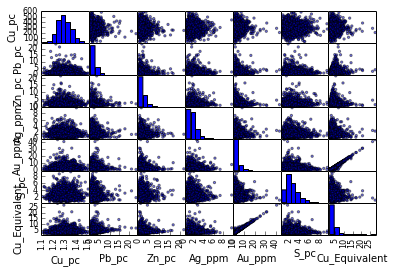Here's an example to make a histogram:

In :
df_assay["Cu_pc"].hist(bins=40, alpha = 0.6)

Out:
<matplotlib.axes.AxesSubplot at 0x610cd30>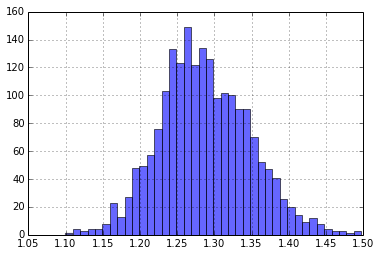Often we will need to plot assay data on a log scale to show anything clearly. Below is an example of how we can modify the Pandas histogram by plotting it on a Matplotlib figure. This gives us the opportunity to change the x axis labels back into real numbers rather than log numbers.

In :
fig, ax = plt.subplots()#define
fig.set_size_inches((10, 6))
np.log10(df_assay["Cu_pc"]).hist(bins=40, ax=ax, alpha = 0.3, color="g")

labels = ax.get_xticks()
new_labels = plt.xticks(labels, np.round(np.power(10, labels),2))
#plt.savefig(output_path + "\\Cu_Hist1.png", dpi = 600)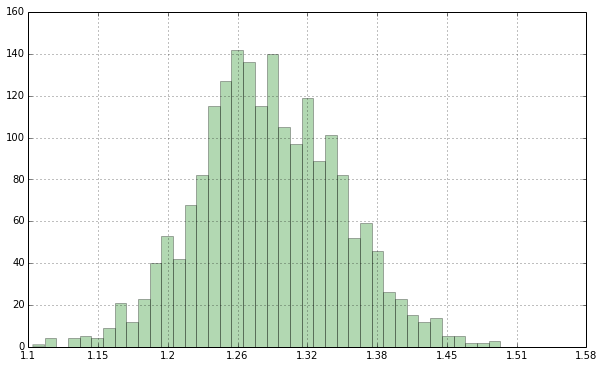Boxplots are also easy and can be grouped by a field in the dataframe. If lists of element field names are supplied seperate subplots will be produced. Groupby fields can also be lists

In :
df_assay.boxplot(column= "Cu_pc", by = ["Zone Code"],  rot = 90)

Out:
<matplotlib.axes.AxesSubplot at 0xbfbe2b0>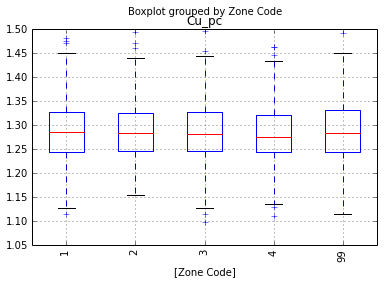Below is an example of the same boxplot on a log scale. The last line (hashed out) saves the figure to the output folder. dpi cpntrols the dots per inch. The higher the dpi the longer it takes to plot and the the larger the file size. I've found that dpi= 600 is about as high as you need to go for putting anything into MS Word. We're using randomly generated data so it doesn't tell us too much but you get the idea.

In :
fig, ax = plt.subplots()#define
fig.set_size_inches((10, 6))
df_assay.boxplot(column= "Cu_pc", by = ["Ox","Zone Code"], ax=ax, rot = 90)
ax.set_yscale('log')
#plt.savefig(output_path + "\\Cu_Boxplot.png", dpi = 600)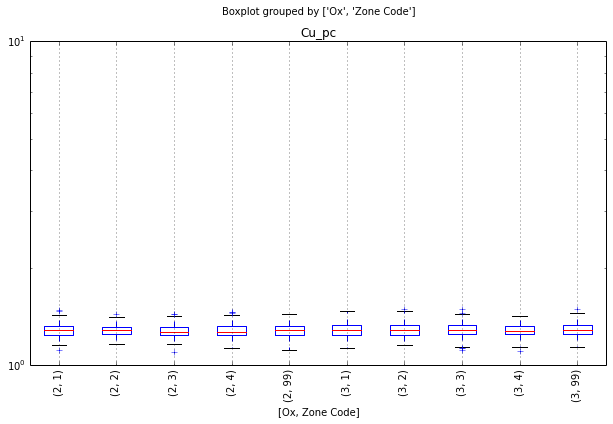If we want to use this plot again and again it's a good idea to put it in a function as in the following. Here we also use the weighted average function that we created earlier to calculate the averages and then plot them on the same graph. The option to save the figure has also been added.

In :
def plot_pandas_bp(df, element_fn, groupby_fns, save_folder= None):
fig, ax = plt.subplots()
fig.set_size_inches((10, 6))
df.boxplot(column= elem, by = groupby_fns, ax=ax, rot = 90)

#Add some weighted averages to the boxplot
wt_means = grouped_weighted_average(df, [element_fn], "Interval", groupby_fns)
x_locations = np.arange(1, len(wt_means)+1,1) # Pandas plots the boxplots on x axis starting from 1
plt.scatter(x_locations, wt_means, color = "red")

ax.set_yscale('log')

# Get rid of the "Boxplot grouped by ..." title
fig.suptitle('')

#Optionally save the the picture
if save_folder is not None:
save_dir = save_folder + "\\Boxplot of " + element_fn + " by " + str(groupby_fns)
plt.savefig(save_dir)


It is then easy to loop through a list like we do here. This will produce a figure for each element in our econ_elems list.

In :
for elem in econ_elems:
plot_pandas_bp(df_assay, elem, ["Ox","Zone Code"])#, save_folder = output_path)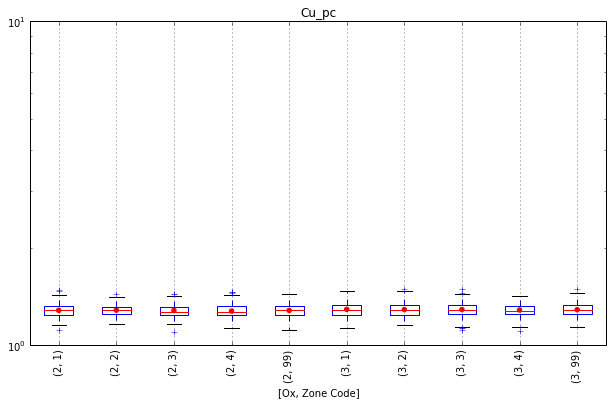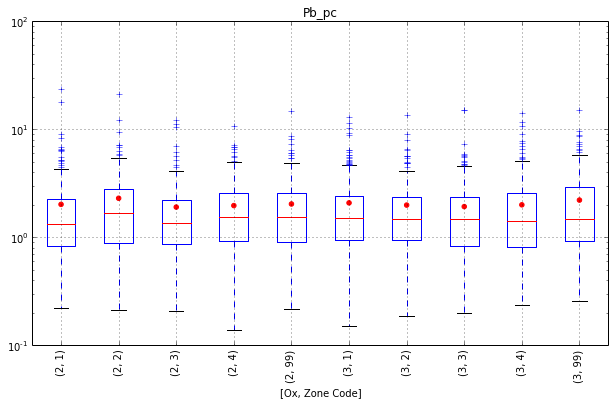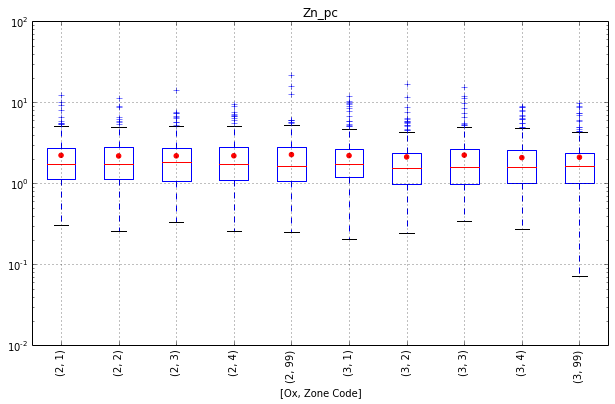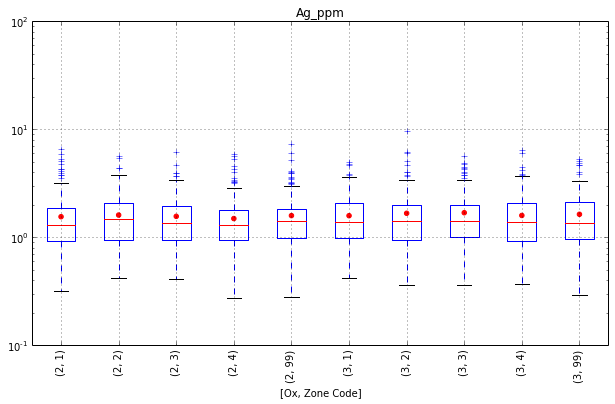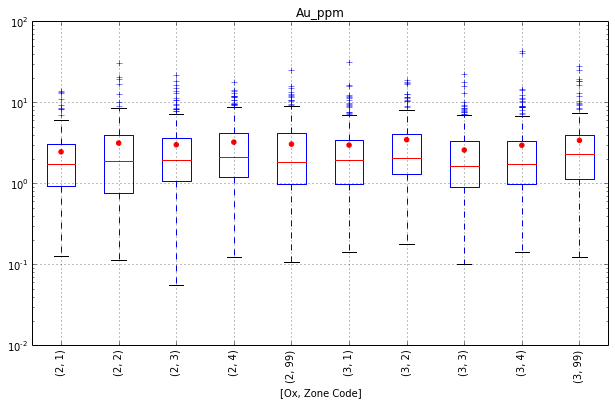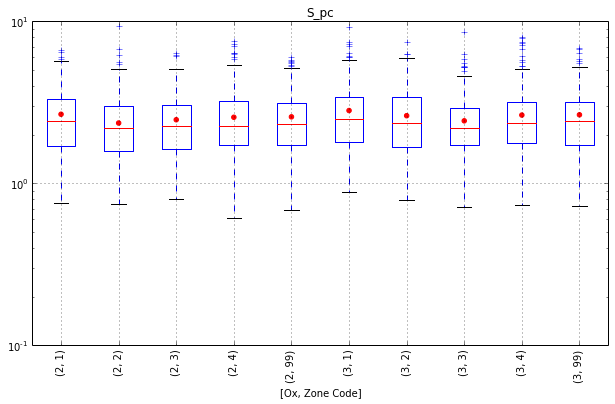One of the things that really bugs me with MS Excel is the amount of faffing around that you need to do to create plots that show multiple series on the same graph. In the following example we create a scatter plot with the help of the pandas groupby function. Here we actually iterate over the grouped data in order to plot it in different colours. We'll put in a function so we can use it later if we want to.

In :
def grouped_scatter(df, x_fn, y_fn, groupby_fns):
fig, ax = plt.subplots()#define
fig.set_size_inches((10, 6))
grouped = df.groupby(groupby_fns)
for group_name, group_data in grouped:
ax.plot(group_data[x_fn], group_data[y_fn], marker='o', linestyle='', ms=8, label=group_name)
ax.set_xlabel(x_fn)
ax.set_ylabel(y_fn)
ax.grid()
legend = ax.legend(numpoints=1, loc= 'best')

In :
grouped_scatter(df_assay, "Cu_pc", "Ag_ppm", "Zone Code")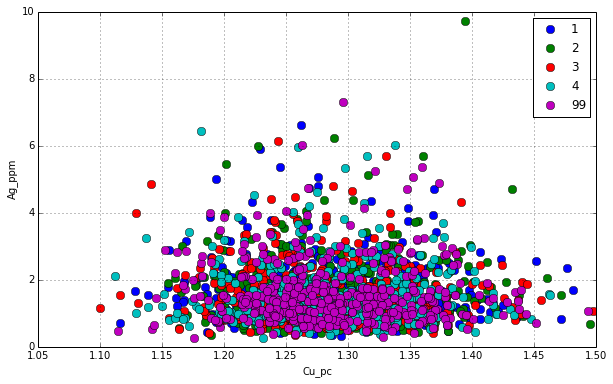# Matplotlib¶

There are loads of examples on the web to copy and paste straight into your code and modify if you want to. The matplotlib gallery is a good place to check out what is available. Here is an example I copied straight out of the gallery (as I'm running out of time!)

In :
"""
http://matplotlib.org/examples/statistics/histogram_demo_multihist.html
Demo of the histogram (hist) function with multiple data sets.

Plot histogram with multiple sample sets and demonstrate:

* Use of legend with multiple sample sets
* Stacked bars
* Step curve with a color fill
* Data sets of different sample sizes
"""
import numpy as np
import matplotlib.pyplot as plt

n_bins = 10
x = np.random.randn(1000, 3)

fig, axes = plt.subplots(nrows=2, ncols=2)
ax0, ax1, ax2, ax3 = axes.flat

colors = ['red', 'tan', 'lime']
ax0.hist(x, n_bins, normed=1, histtype='bar', color=colors, label=colors)
ax0.legend(prop={'size': 10})
ax0.set_title('bars with legend')

ax1.hist(x, n_bins, normed=1, histtype='bar', stacked=True)
ax1.set_title('stacked bar')

ax2.hist(x, n_bins, histtype='step', stacked=True, fill=True)
ax2.set_title('stepfilled')

# Make a multiple-histogram of data-sets with different length.
x_multi = [np.random.randn(n) for n in [10000, 5000, 2000]]
ax3.hist(x_multi, n_bins, histtype='bar')
ax3.set_title('different sample sizes')

plt.tight_layout()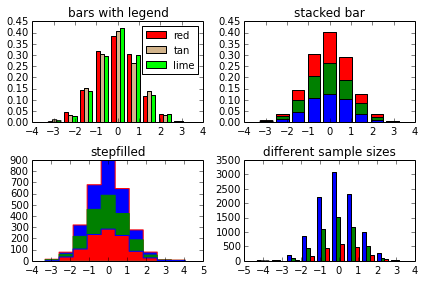It's also easy to share one or both axes. Below is another example I modified from the gallery in five minutes. With a little more work this could turn into a nice way to plot downhole data. At the moment it gives an idea of what is going on downhole. You can see how the data from the Pandas dataframe is provided to the matplotlib plotting.

In :
drill_1 = df_assay[df_assay["hole_id"]== "DH1"]

In :
fig, (ax1, ax2, ax3) = plt.subplots(1,3, sharey=True)#define
fig.set_size_inches((6, 6))

ax1.plot(drill_1["Cu_pc"],drill_1["From"]*-1, c= "r")
ax1.set_xlabel("Cu_pc")
ax2.plot(drill_1["Pb_pc"], drill_1["From"]*-1, c= "b")
ax2.set_xlabel("Pb_pc")
ax3.plot(drill_1["Ag_ppm"], drill_1["From"]*-1, c= "g")
ax3. set_xlabel("Ag_ppm")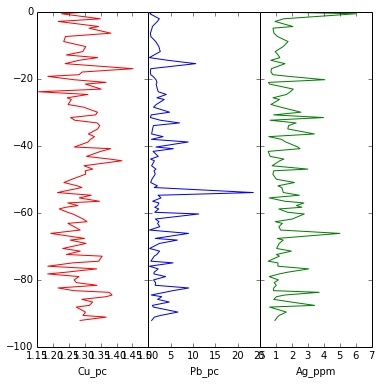With a bit of tweaking you can get some fancy looking graphs. Python makes it easy to automate plotting so you could, for example, write a function to iterate over each drill hole in your table or each standard (CRM) in your QAQC database.

Below is an example that I got from this website by Roger Veciana i Rovira. It uses the Radial Basis Function from Scipy to interpolate grades between points. I added the contour lines and made the points scaled to value size. There are many ways to interpolate between the points so be careful which method you use.

In :
import  scipy.interpolate as interpolate
import matplotlib.pyplot as plt

#Creating some data, with each coordinate and the values stored in separated lists
x = [10,60,40,70,10,50,20,70,30,60]
y = [10,20,30,30,40,50,10,70,80,90]
values = [1,2.2,1,3,4,6,7,7.5,8.3,10]

#Creating the output grid (100x100, in the example)
ti = np.linspace(0, 100.0, 100)
XI, YI = np.meshgrid(ti, ti)

#Creating the interpolation function and populating the output matrix value
rbf = interpolate.Rbf(x, y, values, function='linear') # other functions to try are: 'thin_plate', 'multiquadric,
ZI = rbf(XI, YI)

# Plotting the result
n = plt.Normalize(0.0, 100.0)
fig, ax = plt.subplots()#define
fig.set_size_inches((10, 6.18))
plt.subplot(1, 1, 1)
plt.pcolor(XI, YI, ZI)
plt.scatter(x, y, s = np.array([values])*100, c = values)
plt.colorbar()
CS = plt.contour(XI, YI, ZI, 10, colors='k')
plt.clabel(CS, inline=1, fontsize=10)

plt.title('RBF interpolation')
plt.xlim(0, 100)
plt.ylim(0, 100)

Out:
(0, 100)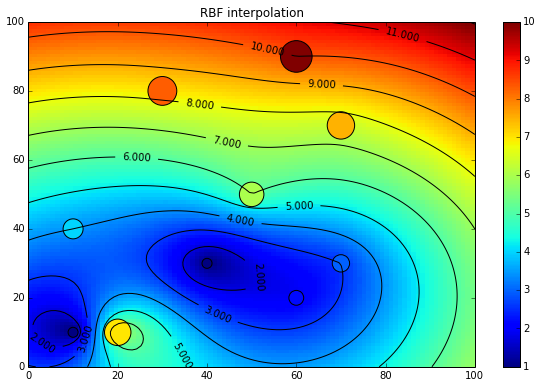# Scipy and Scikit-learn¶

I hope so far the examples have shown you how easy Python is to read and write. In my opinion it beats MS Excel for many simple tasks. Some of the more advanced functions available in Python would be very difficult to achieve in Excel and yet they're not actually any more difficult to apply than the examples we have seen so far.

# Factor Analysis in Scipy¶

When I was preparing for this talk I was planning on demonstrating how easy it is to use Principal Component Analysis (PCA) and apply it to geological applications. This webpage by Sebastian Raschka really helped me understand what PCA does. If you're interested in using PCA it's worth taking the time to go through. It shows a handy trick to help decide how many components you may want to reduce the data to. At the Geochemistry course last week at UNSW I learned that Factor Analysis is more applicable to geological applications. A quick google search told me that Factor Analysis is also available from Scikit-learn and even how it differs from PCA ("PCA assumes equal noise variance for each feature"). Unsurprisingly all the Factor Analysis functions are the same as the PCA functions. If you wanted to test out PCA just change the "decomposition.FactorAnalysis" to "decomposition.PCA".

The following is a example of how to use Factor Analysis and K-Means clustering to take a guess at classifying rock types. Depending on your application you may need to massage the data first to get rid of extreme values and outliers. There are various ways to do this but using Mahalanobis distances may help. This webpage from Scikit-learn shows how it can be applied.

In :
from sklearn import decomposition
from sklearn import cluster
from sklearn import datasets
from sklearn.preprocessing import StandardScaler


First of all we need to select the columns of interest that we want to conduct the classifying on.

In :
cois =['Al_pc', 'Ba_ppm', 'Ca_pc', 'Cr_ppm', 'Fe_pc', 'K_pc', 'Mg_pc', 'Na_pc',  'Ti_pc', 'V_ppm']#'S_pc',


Then we will select these columns and name the dataframe. The dropna() function drops any rows (samples) that do not have a value for every element in the columns of interest. Most (all?) of the Scipy or Scikits-learn functions do not work with missing data. In this example we are left with 6,554 samples.

In :
df_fa = df_assay[cois].dropna()
df_fa.shape

Out:
(2000, 10)

We then need to standardise the data which removes the mean and scales the data to the unit variance.

In :
standardised = StandardScaler().fit_transform(df_fa)


We then set the number of components to look at. In this example we're choosing the seven because I have found that this works well on this dataset. Check out the link to Sebastian Raschka's example in order to work out the eigenvalues for each eigenvector.

We then need to create the Factor Analysis instance and then fit the data and transform it.

In :
n_components = 7
FA = decomposition.FactorAnalysis(n_components= n_components)
Y_FA = FA.fit_transform(standardised)


That's the Factor Analysis completed. Piece of cake! Then we need to classify the data into groups. There are many ways to do this in Scikits-learn. An example of eight different methods is shown near the end of this notebook. Anyway, here we're going to use k-means cluster analysis to group the data into seperate groups. This is an example of unsupervised learning.

First we create the instance and then fit the data. Then we add a column to our dataframe with the labels that the k-means clustering process has assigned to each record.

In :
k_means = cluster.KMeans(init='k-means++', n_clusters= n_components, random_state= 456)
k_means.fit(Y_FA)
df_fa["K_means_Groups"] = k_means.labels_


The next bit isn't actually necessary but I like to do it. k-means will assign labels as numbers from 0 to the n_clusters-1. These numbers are in random order but I prefer to have numbers ascending from 1 to n_clusters (7 here) asecending according to the number of assigned values i.e. the group with the most samples will be group 1 and the group with the least samples will be group 7 in this example. This is also a chance to show another handy Pandas function where we can use a dictionary to find and replace values. Then we take a look at the number of samples assigned to each group.

In :
count_order = df_fa["K_means_Groups"].value_counts().index # Lists the groups in order of counts
dictionary = dict(zip(count_order, range(1, len(count_order)+1))) # Creates a dictionary of groups and the new group names
df_fa["K_means_Groups"].replace(dictionary, inplace = True) # Replaces the groups with the new group names
df_fa["K_means_Groups"].value_counts() # Displays the new group names so we can look at them

Out:
1    437
2    403
3    372
4    301
5    252
6    153
7     82
dtype: int64

Then I want to export the data out again. The problem is that I want all the data back again, not just the columns that are in the df_fa table. This is easy because the df_fa retained the original indexing from the df_assay. We can therefore just use Pandas Join function on the new "K_means_Groups" series (column) and it will automatically put the values in the correct rows. Then export to csv so we can load it into Micromine and take a look.

In :
df_assay_mod = df_assay.join(df_fa[["K_means_Groups"]])
df_assay_mod.to_csv(output_path+"\\Test_kmeans.csv")


You could plot these groups using a series of scatter plots and grouping by the "K_means_Groups" column by modifying one of the examples above to investigate how it has done it's grouping. Here we'll just take a look at the means:

In :
np.round(df_fa.groupby("K_means_Groups").mean(),2)

Out:
Al_pc Ba_ppm Ca_pc Cr_ppm Fe_pc K_pc Mg_pc Na_pc Ti_pc V_ppm
K_means_Groups
1 1.87 2.28 2.83 1.40 1.97 2.70 2.78 2.17 2.26 2.51
2 1.87 2.19 2.72 1.41 2.09 2.03 2.78 1.96 2.27 2.33
3 1.87 2.23 3.20 1.25 2.09 2.25 2.55 2.47 2.25 2.22
4 1.87 2.18 2.61 1.23 1.91 4.12 3.02 2.28 2.23 2.91
5 1.87 2.30 3.05 1.51 1.96 3.11 2.49 2.95 2.28 2.72
6 1.87 2.23 2.75 1.72 1.93 2.94 2.59 2.28 2.31 10.57
7 1.87 2.24 2.69 8.06 2.04 2.55 2.79 2.50 2.25 3.97

Just to get a clearer idea we'll plot a scatter plot grouped by the K-means classification using the grouped_scatter function we created earlier. This doesn't look great using randomly generated data but with real data it can be used to quickly give split rock types based on assay data. I wouldn't trust it implicitly though!

In :
grouped_scatter(df_assay_mod, "Ca_pc", "Na_pc", "K_means_Groups")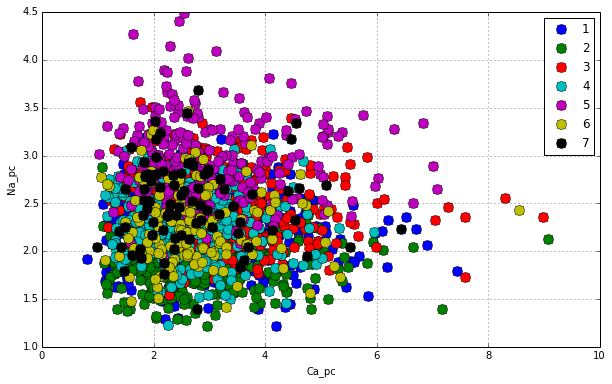If you wanted to get more defination on any of the groups you could repeat the process on each/any of the groups to subdivide them. You'll probably want to change the n_components though.

## Examples of different clustering methods in Scipy¶

Scipy has loads of cluster methods for classification. There are also other ways to classify data. The following example to compare different clustering methods. This webpage gives some backgoud on the different cluster methods and this webpage gives examples of some other classifiers. The code below can be found by clicking on the image on the webpage. I just copied and pasted it below.

In :
import time

import numpy as np
import matplotlib.pyplot as plt

from sklearn import cluster, datasets
from sklearn.neighbors import kneighbors_graph
from sklearn.preprocessing import StandardScaler
import warnings
warnings.filterwarnings('ignore')
np.random.seed(0)

# Generate datasets. We choose the size big enough to see the scalability
# of the algorithms, but not too big to avoid too long running times
n_samples = 1500
noisy_circles = datasets.make_circles(n_samples=n_samples, factor=.5,
noise=.05)
noisy_moons = datasets.make_moons(n_samples=n_samples, noise=.05)
blobs = datasets.make_blobs(n_samples=n_samples, random_state=8)
no_structure = np.random.rand(n_samples, 2), None

colors = np.array([x for x in 'bgrcmykbgrcmykbgrcmykbgrcmyk'])
colors = np.hstack([colors] * 20)

clustering_names = [
'MiniBatchKMeans', 'AffinityPropagation', 'MeanShift',
'SpectralClustering', 'Ward', 'AgglomerativeClustering',
'DBSCAN', 'Birch']

plt.figure(figsize=(len(clustering_names) * 2 + 3, 9.5))
hspace=.01)

plot_num = 1

datasets = [noisy_circles, noisy_moons, blobs, no_structure]
for i_dataset, dataset in enumerate(datasets):
X, y = dataset
# normalize dataset for easier parameter selection
X = StandardScaler().fit_transform(X)

# estimate bandwidth for mean shift
bandwidth = cluster.estimate_bandwidth(X, quantile=0.3)

# connectivity matrix for structured Ward
connectivity = kneighbors_graph(X, n_neighbors=10, include_self=False)
# make connectivity symmetric
connectivity = 0.5 * (connectivity + connectivity.T)

# create clustering estimators
ms = cluster.MeanShift(bandwidth=bandwidth, bin_seeding=True)
two_means = cluster.MiniBatchKMeans(n_clusters=2)
connectivity=connectivity)
spectral = cluster.SpectralClustering(n_clusters=2,
eigen_solver='arpack',
affinity="nearest_neighbors")
dbscan = cluster.DBSCAN(eps=.2)
affinity_propagation = cluster.AffinityPropagation(damping=.9,
preference=-200)

connectivity=connectivity)

birch = cluster.Birch(n_clusters=2)
clustering_algorithms = [
two_means, affinity_propagation, ms, spectral, ward, average_linkage,
dbscan, birch]

for name, algorithm in zip(clustering_names, clustering_algorithms):
# predict cluster memberships
t0 = time.time()
algorithm.fit(X)
t1 = time.time()
if hasattr(algorithm, 'labels_'):
y_pred = algorithm.labels_.astype(np.int)
else:
y_pred = algorithm.predict(X)

# plot
plt.subplot(4, len(clustering_algorithms), plot_num)
if i_dataset == 0:
plt.title(name, size=18)
plt.scatter(X[:, 0], X[:, 1], color=colors[y_pred].tolist(), s=10)

if hasattr(algorithm, 'cluster_centers_'):
centers = algorithm.cluster_centers_
center_colors = colors[:len(centers)]
plt.scatter(centers[:, 0], centers[:, 1], s=100, c=center_colors)
plt.xlim(-2, 2)
plt.ylim(-2, 2)
plt.xticks(())
plt.yticks(())
plt.text(.99, .01, ('%.2fs' % (t1 - t0)).lstrip('0'),
transform=plt.gca().transAxes, size=15,
horizontalalignment='right')
plot_num += 1